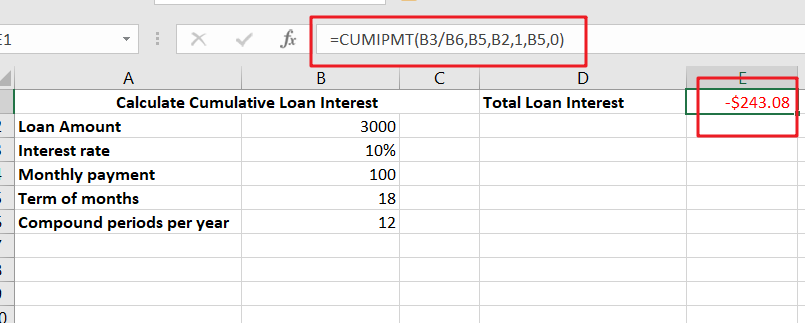# Calculate Cumulative Loan Interest in Excel

## What is Cumulative Loan Interest?

When comparing different types of loans, many people want to know what is Cumulative Loan Interest. The sum of all interest payments you’ve made on a loan is referred to as cumulative interest. Different lenders calculate interest payments differently, but cumulative interest is an important metric when comparing interest costs on two loans.## Calculate Cumulative Loan Interest Excel

If you have a loan that you would like to know the total interest paid over a period of time, you can use the Excel financial function CUMIPMT to calculate this information. You can use the same calculation method for other loans as well.

To calculate the interest rate on loan, you need first to determine how much you have borrowed. A straight-line loan, for example, requires that you pay a fixed amount every period, but interest accrues over time. The Excel function will then return the interest rate for the period. You can also enter example data and use it for practice problems and quizzes. Ultimately, you will need to know the interest rate on loan to determine how much it will cost you.

### Generic Formula:

`=CUMIPMT(rate,nper,pv,start,end,type)`

### Summary:

The formula applied in C10 is:

`=CUMIPMT(B3/B6,B5,B2,1,B5,0)`### Syntax:

• Rate: Interest rate for a specific period
• Nper: Total number of payments
• Start_period: First period of interest
• End_period: last period of interest.

With the given inputs, you can evaluate the total interest paid!

### Clarification:

Excel has a CUMIPMT function that calculates the cumulative loan interest between two periods. It can also compute the total amount of interest paid for a loan. It can calculate the present value of all payments for a certain period and can also determine the type of due date for each payment. It can also be used for comparing the total amount of money owed to a previous loan. The advantages of using Excel for calculating interest on a loan are endless.

### Conclusion:

Using the CUMIPMT function to calculate cumulative loan interest is also helpful. You can specify the number of periods over which you want to calculate the interest and the Start and End periods for which you want to calculate the cumulative interest. This will reveal the amount of cumulative loan interest that will be due over a period of time.